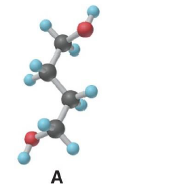# Problem: What is the empirical formula of molecule A below? a. C4H10O4 b. C2H5O2 c. C4H10O2 d. C2H4O e. C2H5O

🤓 Based on our data, we think this question is relevant for Professor Teets' class at UH.

###### Problem Details

What is the empirical formula of molecule A below?

a. C4H10O4

b. C2H5O2

c. C4H10O2

d. C2H4O

e. C2H5OWhat scientific concept do you need to know in order to solve this problem?

Our tutors have indicated that to solve this problem you will need to apply the Empirical Formula concept. You can view video lessons to learn Empirical Formula. Or if you need more Empirical Formula practice, you can also practice Empirical Formula practice problems.

What is the difficulty of this problem?

Our tutors rated the difficulty ofWhat is the empirical formula of molecule A below? a. C4H10...as medium difficulty.

How long does this problem take to solve?

Our expert Chemistry tutor, Sabrina took 2 minutes and 16 seconds to solve this problem. You can follow their steps in the video explanation above.

What professor is this problem relevant for?

Based on our data, we think this problem is relevant for Professor Teets' class at UH.